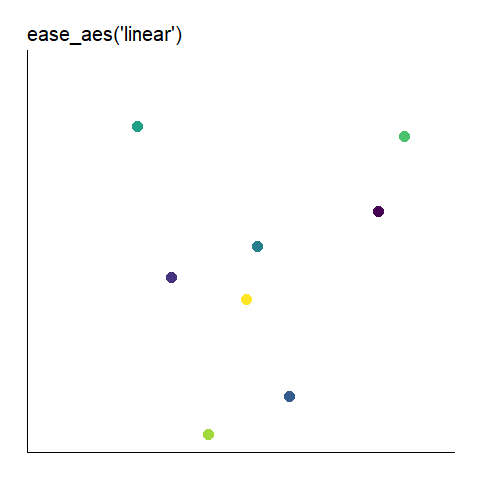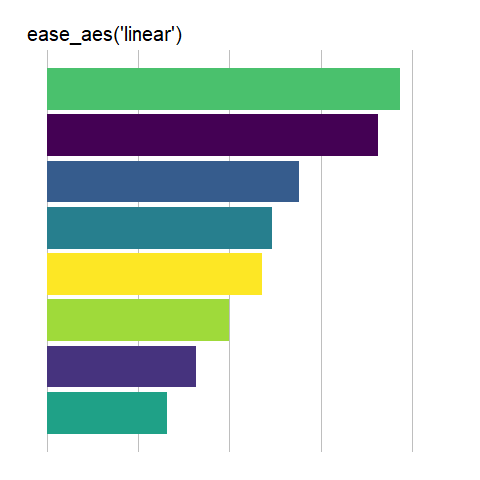Want to share your content on R-bloggers? click here if you have a blog, or here if you don't.

#### Easing

In R, easing is the interpolation, or tweening, between successive states of a plot (1). It is used to control the motion of data elements in animated data displays (2), with different easing functions giving different appearances or dynamics to the display’s animation.

#### The ease_aes() Function

The ease_aes() function controls the easing of aesthetics or variables in gganimate. The default, ease_aes(), models a linear transition between states. Other easing functions are specified using the easing function name, appended with one of three modifiers (3) :
###### Easing Functions
quadratic models an exponential function of exponent 2.
cubic models an exponential function of exponent 3.
quartic models an exponential function of exponent 4.
quintic models an exponential function of exponent 5.
sine models a sine function.
circular models a pi/2 circle arc.
exponential models an exponential function of base 2.
elastic models an elastic release of energy.
back models a pullback and release.
bounce models the bouncing of a ball.
###### Modifiers
-in applies the easing function as-is.
-out applies the easing function in reverse.
-in-out applies the first half of the transition as-is and the last half in  reverse.

The formulas used to implement these options can be found here (4).  They are illustrated below using animated scatter plots and bar charts.

#### Data File Description

‘data.frame’: 40 obs. of 7 variables:
Cat : chr “A” “A” “A” “A” …
OrdInt: int 1 2 3 4 5 1 2 3 4 5 …
X : num 70.5 78.1 70.2 78.1 70.5 30.7 6.9 26.7 6.9 30.7 …
Y : num 1.4 7.6 -7.9 7.6 1.4 -7 -23.8 19.8 -23.8 -7 …
Rank : int 2 2 2 2 2 7 8 8 8 7 …
OrdDat: chr “01/01/2019” “04/01/2019” “02/01/2019” “05/01/2019” …
Ord : Date, format: “2019-01-01” “2019-04-01” “2019-02-01” “2019-05-01” …

The data used for this demo is a much abbreviated and genericized version of  this (5) data set.

#### Scatter Plots

Here is the code used for the ease_aes(‘cubic-in’) animated scatter plot:
```# load libraries
library(gganimate)
library(tidyverse)

# the data file format is indicated above

# convert date to proper format
data\$Ord <- as.Date(data\$OrdDat, format='%m/%d/%Y')
# specify the animation length and rate
options(gganimate.nframes = 30)
options(gganimate.fps = 10)

# loop the animation
options(gganimate.end_pause = 0)
# specify the data source and the X and Y variables
ggplot(data, aes(X, Y)) +

# specify the plot format
theme(panel.background = element_rect(fill = 'white'))+
theme(axis.line = element_line()) +
theme(axis.text = element_blank())+
theme(axis.ticks = element_blank())+
theme(axis.title = element_blank()) +
theme(plot.title = element_text(size = 20)) +
theme(plot.margin = margin(25, 25, 25, 25)) +
theme(legend.position = 'none') +

# create a scatter plot
geom_point(aes(color = Cat), size = 5) +

# indicate the fill color scale
scale_fill_viridis_d(option = "D", begin = 0, end = 1) +

# apply the fill to the 'Cat' variable
aes(group = Cat) +

# animate the plot on the basis of the 'Ord' variable
transition_time(Ord) +

# the ease_aes() function
ease_aes('cubic-in') +

# title the plot
labs(title = "'ease_aes(cubic-in)'")
```This is the default value, equivalent to ease_aes(). It is the only easing function that does not take a modifier.

#### Bar Charts

Here is the code used for the ease_aes(‘cubic-in’) animated bar chart:
```# load libraries
library(gganimate)
library(tidyverse)

# the data file format is indicated above

# convert date to proper format
data\$Ord <- as.Date(data\$OrdDat, format='%m/%d/%Y')

# specify the animation length and rate
options(gganimate.nframes = 30)
options(gganimate.fps = 10)

# loop the animation
options(gganimate.end_pause = 0)

# specify the data source
ggplot(data) +

# specify the plot format
theme(panel.background = element_rect(fill = 'white'))+
theme(panel.grid.major.x  = element_line(color='gray'))+
theme(axis.text = element_blank())+
theme(axis.ticks = element_blank())+
theme(axis.title = element_blank()) +
theme(plot.title = element_text(size = 20)) +
theme(plot.margin = margin(25, 25, 25, 25)) +
theme(legend.position = 'none') +

# specify the x and y plot limits
aes(xmin = 0, xmax=X+2) +
aes(ymin = Rank-.45,
ymax = Rank+.45,
y = Rank) +

# create a bar chart
geom_rect() +

# indicate the fill color scale
scale_fill_viridis_d(option = "D", begin = 0, end = 1) +

# place larger values at the top
scale_y_reverse() +

# apply the fill to the 'Cat' variable
aes(fill = Cat) +

# animate the plot on the basis of the 'Ord' variable
transition_time(Ord) +

# the ease_aes() function
ease_aes('cubic-in') +

# title the plot
labs(title = "'ease_aes(cubic-in)'")

```This is the default value, equivalent to ease_aes(). It is the only easing function that does not take a modifier.

#### Other Resources

Here (6) is a nice illustration of the various easing options presented as animated paths.

Here (7) and here (8) are articles presenting various animated plots using ease_aes().

Here (9) are some other animations using ease_aes().

#### References

1 https://www.rdocumentation.org/packages/gganimate/versions/1.0.7/topics/ease_aes

2 https://rdrr.io/cran/tweenr/

3 https://gganimate.com/reference/ease_aes.html

4 https://github.com/thomasp85/tweenr/blob/master/src/easing.c

6 https://easings.net/

7 https://github.com/ropenscilabs/learngganimate/blob/master/ease_aes.md

8 https://www.statworx.com/de/blog/animated-plots-using-ggplot-and-gganimate/

9 https://www.r-graph-gallery.com/animation.html

ease_aes() Demo was first posted on January 15, 2021 at 8:45 am.